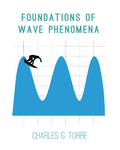## Foundations of Wave Phenomena

#### Title

12 Cylindrical Coordinates

#### Description

We have seen how to build solutions to the wave equation by superimposing plane waves with various choices for amplitude, phase and wave vector k. In this way we can build up solutions which need not have the plane symmetry (exercise), or any symmetry whatsoever. Still, as you know by now, many problems in physics are fruitfully analyzed when they are modeled as having various symmetries, such as cylindrical symmetry or spherical symmetry. For example, the magnetic field of a long, straight wire carrying a steady current can be modeled as having cylindrical symmetry. Likewise, the sound waves emitted by a pointlike source are nicely approximated as spherically symmetric. Now, using the Fourier expansion in plane waves we can construct such symmetric solutions — indeed, we can construct any solution to the wave equation. But, as you also know, we have coordinate systems that are adapted to a variety of symmetries, e.g., cylindrical coordinates, spherical polar coordinates, etc. When looking for waves with some chosen symmetry it is advantageous to get at the solutions to the wave equation directly in these coordinates, without having to express them as a superposition of plane waves. Our task now is to see how to express solutions of the wave equations in a useful fashion in terms of such curvilinear coordinate systems.

8-2014

#### Keywords

Cylindrical Coordinates, fourier expansion, chapter 12

#### Disciplines

Physical Sciences and Mathematics | Physics